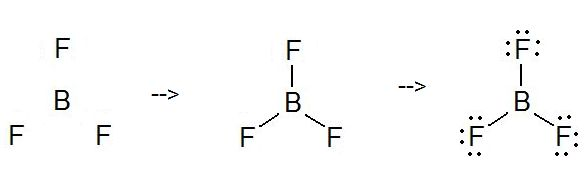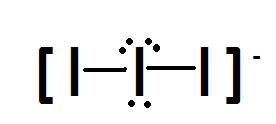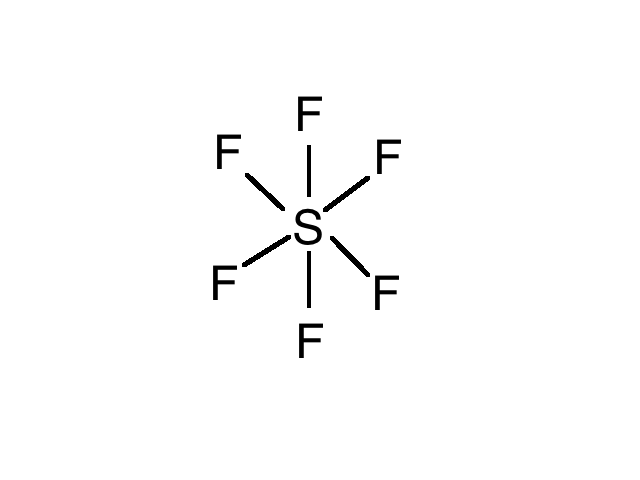# 2.1B: Lewis Structures

Lewis Structures are very similar to electron dot diagrams except for the fact that shared electrons between atoms are shown as lines. Lone pairs of electrons themselves are usually represented by dots around the atoms. These diagrams are helpful because they allow us to predict the shape of the molecule and see the positions of each atom relative to the others.

## Introduction

In 1916, Gilbert Lewis Newton introduced a way to show the bonding of atoms in a molecule though diagrams. Creating Lewis Structures is rather simple and only requires a small series of steps and little bit of math.

## Basic Steps for Simple Structures

Lewis Structure are very simple to construct and only take a few short steps. Make sure to have a paper and pencil handy because you will be drawing these molecules, as well as doing some simple math.

For this module, we will use Boron trifluoride as our example.

1. First, you write the the formula using atomic symbols, BF3. The next step requires you to pick the least electronegative atom to be your central atom. In this case, boron (B) will be our central atom.
2. Next, you must sum of the total amount of valence electrons in the outer most orbital of each atom. Boron contains 3 valence electrons while the fluorine atoms contain 21 valence electrons (7 each). The total amount of electrons we have to work with is 3+21= 24 electrons. In some cases, there may be a charge on the molecule you are working on. If this charge is negative, then you add the value of that charge to the amount of electrons you totaled up. Whereas if the charge is positive, then you subtract that value from the amount that you totaled up. In this case, we do not have a charge on our molecule, so we will stick to using 24 electrons.
3. The next step requires some drawing. Draw out your central atom and place the three fluorine atoms a small distance away from it. The fluorine molecules should be an equal distance away from each other.
4. Next, you must draw a short line connecting the boron atom to each of the fluorine atom. These short lines each represent 2 electrons that are being shared between the two bonded atoms. Because we have 3 lines in this diagram, it means that we have used up 6 of our original 24 electrons, leaving us with 18 electrons left. These 18 electrons will be used to fill the octets of the atoms surrounding the central atom. These electrons will be known as the lone pairs because they are not bonded to another atom. You will represent these electrons by using dots around the atom, each dot represents one electrons. Because each fluorine requires another 6 electrons each to fill their octets, this means that we have used up all of our remaining electrons. Boron does not fill its octet because it is one of the few exceptions to the rule. It is perfectly fine only forming 3 bonds.

The final structure can be seen in a diagram below. Because there are three bonded atoms to a central atom with no lone pairs on it, be can also conclude that BF3 has a trigonal planar geometry with sp2 hybridization.BF3 is only one of the many simple Lewis Structures. There are others that require a little bit more time in creating because they contain a little bit more criteria to complete it. Some of the more complex structures may contain charges as well as double or triple bonds. There are also many molecules that break the octet rule and can form a total of 5 or 6 bonds to the central atom.

## Formal Charges

When drawing Lewis Structures you need to check the structure's formal charge. Unless a charge is preferred you want the overall charge of the molecule to be 0.

Formal Charge = # of valence electrons - # of unpaired electrons - (1/2) # of paired electrons.

So the formal charges are:

FCBoron = 3-0-(1/2)6 = 0

FCFluorine = 7-6-(1/2)2 = 0

FCTotal = FCBoron + FCFluorine = 0 + 0 = 0

If a formal charge is preferred, then the final answer should be in brackets with the charge outside of the bracket.

## Lewis structures of Charged Molecules

In the case of Triiodide (I3-), you notice that there is a negative charge. That means that instead of the 21 valence electrons (7 for each I), you add one more electron to make it 22. You will then create the structure though the steps that were given above. The only additional step required is to put your final structure in brackets with the charge of the molecule outside of the brackets. Triiodide can be seen below.## Multiple Bonds in Lewis Strutures

In the case of CO2, when it comes time to create a lewis structure, it seems as though you don not have enough electrons to complete the octets of all the atoms. When you run into this problem, it usually means that the molecule will contain a double or triple bond.

There are 16 valence electrons in a CO2 molecule. If you go about the steps that were given to you above, you will notice that the octets of the oxygen atoms have been filled, but the carbon still needs 4 more electrons. What you need to do it move one pair of electrons from each oxygen atom and turn it into a double bond on each side of the carbon. The final structure for CO2 can be seen below.## Problems

Complete the following problems to to evaluate your understanding of this module:

Draw the Lewis structure for the following molecules:

1. NO2+

2. C2H6

3. SF6

Find the Formal Charge on all atoms of:

4. NO2+

5. C2H6

6. SF6

1. 2. 3.4. FCN = 5-0-(0.5)8 = +1

FCO = 6-4-(0.5)4 = 0

5. FCC =4-0-(0.5)8 = 0

FCH = 1-0-(0.5)2 = 0

6. FCS = 6-0-(0.5)12 = 0

FCF = 7-6-(0.5)2 = 0

## References

1. Housecroft, Catherine E., and Alan G. Sharpe. Inorganic Chemistry. 3rd ed. Harlow: Pearson Education, 2008. Print.
2. Wade, L. G. Organic Chemistry. 7th ed. Upper Saddle River, NJ: Pearson Prentice Hall, 2010. Print.
3. Shagoury, Richard. Chemistry 1A Lecture Book. 4th Ed. Custom Publishing. 2006. Print